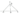# Introduction to Signals MCQ

## Introduction to Signals MCQ

Introduction to Signals MCQ, Signals and Systems MCQ, Multiple Choice Questions on Signals, Energy Signal MCQ, Power Signal MCQ, Engineering MCQ, Electronics MCQ,

Introduction to Systems MCQ

### Multiple Choice Questions

Q.1. Which of the following signals is/are periodic?

• s(t)=\cos 2t+\cos 3t+\cos 5t
• s(t)=\exp (j8\Pi t)
• s(t)=e^{-7t}\sin 10\Pi t
• s(t)=\cos 2t \cos tu(t)

Answer: s(t)=\cos 2t+\cos 3t+\cos 5t & s(t)=\exp (j8\Pi t)

Q.2. Let δ(t) denote the delta function. The value of the integral .1.7,6(t)cos —2 dt is

• 1
• -1
• 0
• π/2

Q.3. If a signal f(t) has energy E, the energy of the signal f (2t) is equal to

• E
• E/2
• 2E
• 4E

Q.4. The function x(t) is shown in the figure. Even and odd parts of a unit-step function u(t) are respectively

• \frac{1}{2}, \frac{1}{2}x(t)
• -\frac{1}{2}, \frac{1}{2}x(t)
• \frac{1}{2}, -\frac{1}{2}x(t)
• None of the above

Q.5. The period of the function \cos \frac{\Pi }{4}(t-1) is

• \frac{1}{8}sec
• 8 sec
• 4 sec
• \frac{1}{4}sec

Q.6. A signal x(t)=A\cos (\Omega _{0}t+\phi ) is

• An energy signal
• A power signal
• An energy as well as a power signal
• Neither an energy nor a power signal

Q.7. x_{1}(t)=2\sin \Pi t+\cos 4\Pi t and x_{2}(t)=\sin 5\Pi t+3\sin 3\Pi t then

• x1 and x2 both are periodic.
• x1 and x2 both are not periodic.
• x1 is periodic, but x2 is not periodic.
• x1 is not periodic, but x2 is periodic.

Answer: x1 and x2 both are periodic.

Q.8. The signal x(t)=2(\cos \Pi t)+3\sin (2t)

• is periodic
• is not periodic
• may or may not be periodic
• none of the above

Q.9. The odd and even components of signal u(t) are

• \cos t , \sin t
• \sin t , -\cos t
• \cos t ,j \sin t
• \cos t ,-j \sin t

Answer: \cos t ,j \sin t

Q.10. The odd and even components of the sequence

• \left\{ 0.25,0,-0.25\right\},\left\{0.75,1,0.75 \right\}
• \left\{ 1.5,0,-1.5\right\},\left\{1.5,1,1.5 \right\}
• \left\{ 0.25,0,-0.25\right\},\left\{0.5,1,0.5 \right\}
• \left\{ 0.25,0,-0.25\right\},\left\{1.5,1,1.5 \right\}

Q.11. The signal x(t)=e^{-2t}u(t) is

• power signal
• energy signal
• neither power nor energy signal
• either power or energy signal

Q.12. The signal x(n)=\cos \frac{2\Pi }{3}nu(n)  is

• causal, aperiodic
• causal, periodic
• non causal periodic
• non-causal aperiodic

Q.13. The value of the integral \int_{-\infty }^{\infty }\delta (at-b)=

• \frac{1}{a^{2}}
• \frac{1}{\left| a\right|}
• \frac{1}{a}
• -\frac{1}{a}

Q.14. The time period of the signal x(t)=\cos 2\Pi t + \sin 5\Pi t is

• 2 sec
• 5 sec
• 10 sec
• 2.5 sec

Q.15. The signal x(n)=\cos 2n is

• periodic with period π
• periodic with period 2π
• periodic with period 4π
• aperiodic

Q.16. Consider the following signals x(t) and y(t ). The relation between x(t) and y(t) can be y(t)

• x(t+1)
• x(2t+2)
• x(t-1)
• x(2t-1)

Q.17. Represent the following signal as the sum of singularity functions

• -r(t)+2r(t)u(t-1)
• r(-t+1)+r(t-1)+2u(t-2)-r(t-2)
• r(-t+1)u(t)+2r(t-1)-r(t-2)-u(t-2)
• r(-t+1)u(t)+r(t-1)-r(t-2)-u(t-2)

Answer: